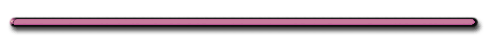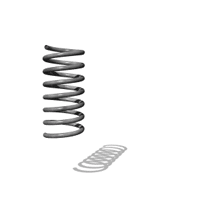Euler's disk

What energy change occurs below?

II. Energy (Joules)

- 2 kinds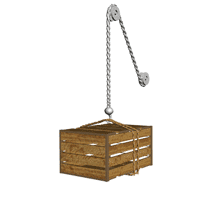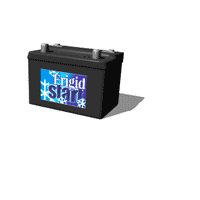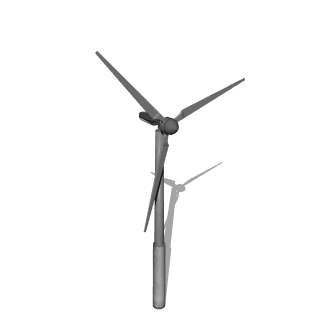Potential & Kinetic Energy

A) Potential Energy - stored energy that an object has due to its position or condition.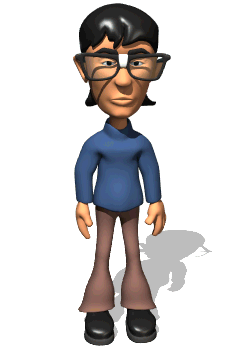"At what position(s) or condition(s) do these  objects have the most PE?"

1. Gravitational P.E.g

(PE = Potential Energy)Two factors determine PE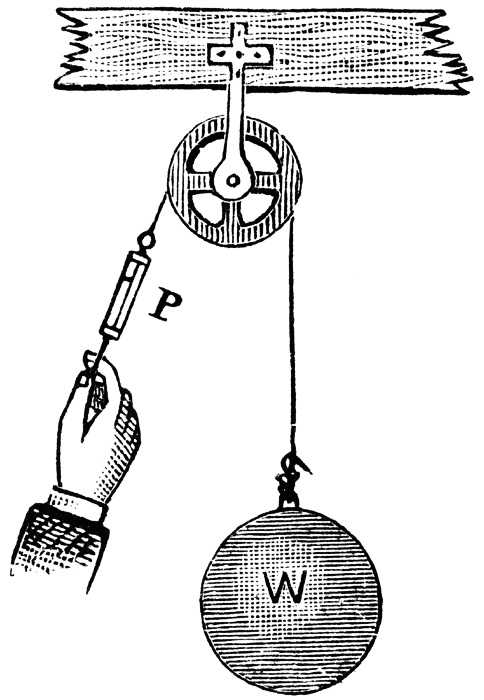Source: Elroy M. Avery School Physics (New York: Sheldon and Company, 1895) 141

Height, weight

 P.E.g  = (mg)h = (weight)h Joules Kg (m/s2)m N m (Not in reference)

g on earth = 9.8 m/s2

mg = weight

(force it could exert)h = height

(distance force could be exerted)

 ΔP.E. = mgΔh = wΔh (Not in reference)

 Ex 1) What P.E. is gained when a 100. kg object is raised 4.00 m straight up?Ex 1) What P.E. is gained when a 100. kg object is raised 4.00 m straight up?

m = 100. kg

h = 4.00 m

ΔP.E. = mgΔh

= (100. kg)(9.81 m/s2)4.00 m

= 3920 J

 Ex 2) What PE would be  gained if an object were  moved 4 m to the right?

ΔP.E. = 0Ex 3) An object weighing 15. N is lifted from the ground to a height of .22 m. Find object's change in PE

Ex 3) An object weighing 15. N is lifted from the ground to a height of 0.22 m. Find object's change in PE

 ΔP.E. = mgΔh = wΔh (Not in reference)

w = 15. N

h = .22 m

ΔP.E. = wΔh

ΔP.E. = 15. N(.22m)

ΔP.E. = 3.3 Nm"Crow bars reduce the force needed to move a body"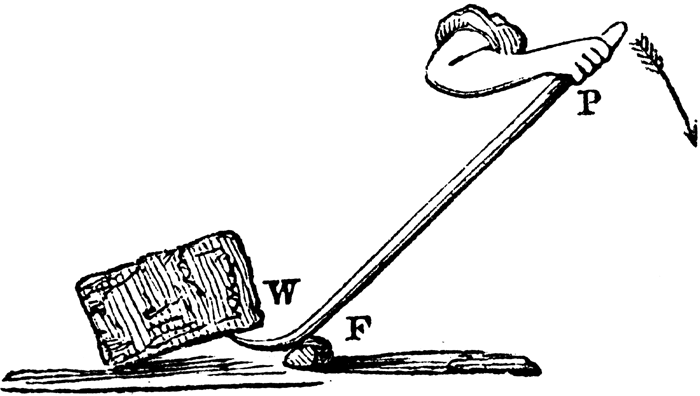G. P. Quackenbos A.M. A Natural Philosophy: Embracing the Most Recent Discoveries in the Various Branches of Physics, and Exhibiting the Application of Scientific Principles in Every-day Life (New York: D. Appleton and Company, 1859) 95

Clipart ETC"... remember what you learned on a seesaw? "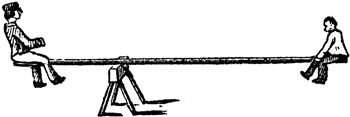Clipart ETC

Work = DPE

W + F & D same direction

W - F & D opp. direction

Ex) A 1.60 m person lifts a 2.10 kg book from the ground to 2.20 m above the ground.

a) How much work was done lifting the book?

Work = DPE

W = mgDh

Work = 2.10kg[9.80 m/s2]2.20m

W = 4.5.3 J

c) How much W is done relative to ground? / relative to top of head/- AP